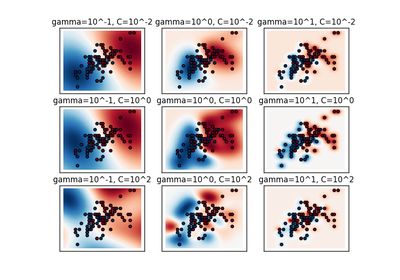# `sklearn.model_selection`.StratifiedShuffleSplit¶

class `sklearn.model_selection.``StratifiedShuffleSplit`(n_splits=10, test_size=0.1, train_size=None, random_state=None)[source]

Stratified ShuffleSplit cross-validator

Provides train/test indices to split data in train/test sets.

This cross-validation object is a merge of StratifiedKFold and ShuffleSplit, which returns stratified randomized folds. The folds are made by preserving the percentage of samples for each class.

Note: like the ShuffleSplit strategy, stratified random splits do not guarantee that all folds will be different, although this is still very likely for sizeable datasets.

Read more in the User Guide.

Parameters: n_splits : int (default 10) Number of re-shuffling & splitting iterations. test_size : float (default 0.1), int, or None If float, should be between 0.0 and 1.0 and represent the proportion of the dataset to include in the test split. If int, represents the absolute number of test samples. If None, the value is automatically set to the complement of the train size. train_size : float, int, or None (default is None) If float, should be between 0.0 and 1.0 and represent the proportion of the dataset to include in the train split. If int, represents the absolute number of train samples. If None, the value is automatically set to the complement of the test size. random_state : int or RandomState Pseudo-random number generator state used for random sampling.

Examples

```>>> from sklearn.model_selection import StratifiedShuffleSplit
>>> X = np.array([[1, 2], [3, 4], [1, 2], [3, 4]])
>>> y = np.array([0, 0, 1, 1])
>>> sss = StratifiedShuffleSplit(n_splits=3, test_size=0.5, random_state=0)
>>> sss.get_n_splits(X, y)
3
>>> print(sss)
StratifiedShuffleSplit(n_splits=3, random_state=0, ...)
>>> for train_index, test_index in sss.split(X, y):
...    print("TRAIN:", train_index, "TEST:", test_index)
...    X_train, X_test = X[train_index], X[test_index]
...    y_train, y_test = y[train_index], y[test_index]
TRAIN: [1 2] TEST: [3 0]
TRAIN: [0 2] TEST: [1 3]
TRAIN: [0 2] TEST: [3 1]
```

Methods

 `get_n_splits`([X, y, groups]) Returns the number of splitting iterations in the cross-validator `split`(X, y[, groups]) Generate indices to split data into training and test set.
`__init__`(n_splits=10, test_size=0.1, train_size=None, random_state=None)[source]
`get_n_splits`(X=None, y=None, groups=None)[source]

Returns the number of splitting iterations in the cross-validator

Parameters: X : object Always ignored, exists for compatibility. y : object Always ignored, exists for compatibility. groups : object Always ignored, exists for compatibility. n_splits : int Returns the number of splitting iterations in the cross-validator.
`split`(X, y, groups=None)[source]

Generate indices to split data into training and test set.

Parameters: X : array-like, shape (n_samples, n_features) Training data, where n_samples is the number of samples and n_features is the number of features. Note that providing `y` is sufficient to generate the splits and hence `np.zeros(n_samples)` may be used as a placeholder for `X` instead of actual training data. y : array-like, shape (n_samples,) The target variable for supervised learning problems. Stratification is done based on the y labels. groups : object Always ignored, exists for compatibility. train : ndarray The training set indices for that split. test : ndarray The testing set indices for that split.

## Examples using `sklearn.model_selection.StratifiedShuffleSplit`¶RBF SVM parameters